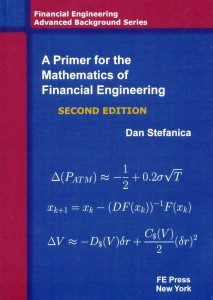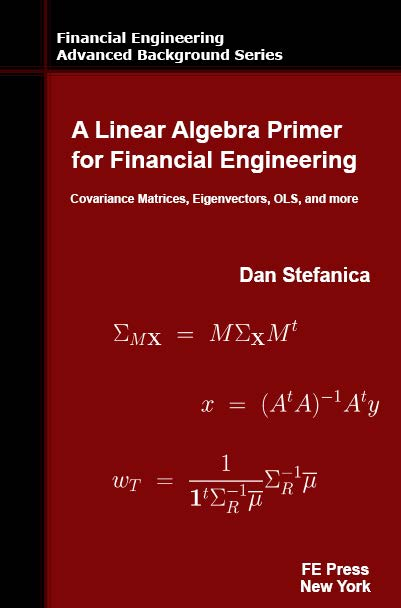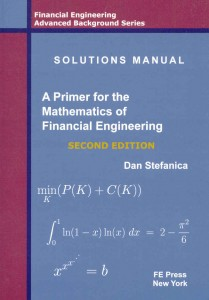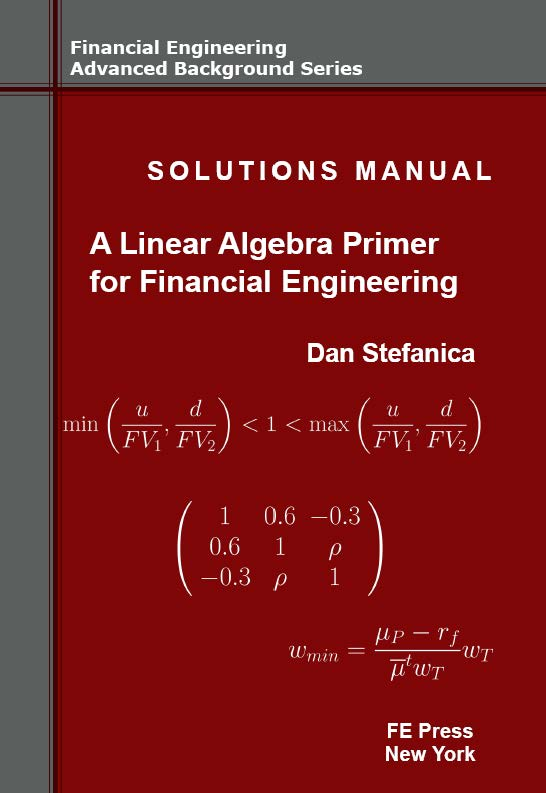# 150 Most Frequently Asked Questions on Quant Interviews#### FE Press offers 15-25% discounts off the list price when two or more books are purchased together. All the books purchased from FE Press are signed and personalized by the authors.

The book “150 Most Frequently Asked Questions on Quant Interviews” is the Number 1 QuantNet bestselling book of 2016, and was the Number 1 QuantNet bestselling book in 2015 and Number 2 in 2014.

Titles Price ORDER• A Primer for the Mathematics of Financial Engineering
• A Linear Algebra Primer for Financial Engineering• A Primer for the Mathematics of Financial Engineering
• A Linear Algebra Primer for Financial Engineering
• 150 Most Frequently Asked Questions on Quant Interviews• A Primer for the Mathematics of Financial Engineering
• Solutions Manual – A Primer for the Mathematics of Financial Engineering
• A Linear Algebra Primer for Financial Engineering
• 150 Most Frequently Asked Questions on Quant Interviews• A Primer for the Mathematics of Financial Engineering
• Solutions Manual – A Primer for the Mathematics of Financial Engineering• A Primer for the Mathematics of Financial Engineering
• Solutions Manual – A Primer for the Mathematics of Financial Engineering
• A Linear Algebra Primer for Financial Engineering• A Primer for the Mathematics of Financial Engineering
• Solutions Manual – A Primer for the Mathematics of Financial Engineering
• 150 Most Frequently Asked Questions on Quant Interviews• A Primer for the Mathematics of Financial Engineering
• 150 Most Frequently Asked Questions on Quant Interviews• A Linear Algebra Primer for Financial Engineering
• 150 Most Frequently Asked Questions on Quant Interviews

150 Most Frequently Asked Questions on Quant Interviews is the first book in the Pocket Book Guides for Quant Interviews Series and contains over 150 questions that are frequently, and also currently, asked on interviews for quantitative positions, covering a vast spectrum, from C++ and data structures, to finance, stochastic calculus and brainteasers.

Topics:

• Mathematics, calculus, differential equations
• Covariance and correlation matrices. Linear algebra
• Financial instruments: options, bonds, swaps, forwards, futures
• C++, algorithms, data structures
• Monte Carlo simulations. Numerical methods
• Probability. Stochastic calculus
• Brainteasers

The authors have over 20 years of experience working with the students of the Baruch College Financial Engineering Masters Program who have consistently been successful interviewing for quant type positions in the New York job market, with over 90% placement every year, including through the challenging 2008-2009 years.

Dan Stefanica has been the Director of the Baruch MFE Program since its inception in 2002, and is the author of the best-selling A Primer For The Mathematics Of Financial Engineering, now in its second edition. He teaches graduate courses on numerical methods for financial engineering, as well as pre-program courses on advanced calculus and numerical linear algebra with financial applications. His research spans numerical analysis, graph theory, and geophysical fluid dynamics. He has a PhD in mathematics from New York University and taught previously at the Massachusetts Institute of Technology.

Rados Radoicic has been on the faculty of the Baruch MFE Program since 2006, teaching graduate courses on financial instruments, econometrics, and statistics, as well as pre-program courses on advanced calculus with financial applications. He has done extensive research in discrete and computational geometry, extremal combinatorics, and graph theory. He has a BS and a PhD in mathematics from the Massachusetts Institute of Technology.

Tai-Ho Wang has been on the faculty of the Baruch MFE Program since 2008, teaching graduate courses on stochastic processes and optimization methods, as well as pre-program courses in probability. His research spans fields as varied as quantitative finance, statistics, and Riemannian geometry. He has a PhD in applied mathematics from National Chiao Tung University.

Errata as of November 16, 2013.

Book Details:

• Softcover: 224 pages
• Publisher: Financial Engineering Press
• List Price: \$29.50
• ISBN-13: 978-0979757648
• ISBN-10: 0979757649

List Price: 29.50

Titles from FE Press:A Linear Algebra Primer for Financial Engineering, by Dan Stefanica, FE Press 2014. This book covers linear algebra concepts for financial engineering applications from a numerical point of view. The book contains many such applications, as well as pseudocodes, numerical examples, and questions often asked in interviews for quantitative positions. Table of Contents. This is the third book in the Financial Engineering Advanced Background Series.150 Most Frequently Asked Questions on Quant Interviews, by Dan Stefanica, Rados Radoicic, and Tai-Ho Wang. FE Press, 2013. This book contains over 150 questions that are frequently, and also currently, asked on interviews for quantitative positions, covering a vast spectrum, from C++ and data structures, to finance, stochastic calculus and brainteasers. A ten questions selection, with solutions, can be downloaded here. This is the first book in the Pocket Book Guides for Quant Interviews Series.“A Primer for the Mathematics of Financial Engineering”, Second Edition, by Dan Stefanica. FE Press, 2011. This book builds the solid mathematical foundation required to understand the quantitative models used financial engineering and can be used as a reference book or as a self-study book. It contains 175 exercises, many of these being frequently asked interview questions. A Solutions Manual including detailed solutions to every exercise was published concurrently. The Second Edition of A Primer for the Mathematics of Financial Engineering was the Number 1 QuantNet bestselling book of 2013. This is the first book in the Financial Engineering Advanced Background Series.Solutions Manual – A Primer for the Mathematics of Financial Engineering, Second Edition, by Dan Stefanica. FE Press, 2011. 2012. Top 3 QuantNet bestselling book in 2010, 2011, 2012, and 2013. This is the second book in the Financial Engineering Advanced Background Series.

# A Primer for the Mathematics of Financial Engineering, Second Edition

#### FE Press offers 15-25% discounts off the list price when two or more books are purchased together. All the books purchased from FE Press are signed and personalized by the authors.

The Second Edition of the A Primer for the Mathematics of Financial Engineering is the Number 3 QuantNet bestselling book of 2015, and was the Number 1 QuantNet bestselling book in 2014, 2013, 2012, 2011.“A Primer for the Mathematics of Financial Engineering” builds the solid mathematical foundation required to understand the quantitative models used financial engineering and can be used as a reference book or as a self-study book. It contains 175 exercises, many of these being frequently asked interview questions. A Solutions Manual including detailed solutions to every exercise was published concurrently.

Sample Sections:

• 8.6. Integrating the density function of the standard normal variable (Download)

Book Details: 2nd Edition; 352 pages; List Price: \$62; Financial Engineering Press, 2011

Dan Stefanica has been the Director of the Financial Engineering Masters Program at Baruch College, City University of New York, since its inception in 2002, and is the author of the best-selling “A Primer For The Mathematics Of Financial Engineering” and of “A Linear Algebra Primer for Financial Engineering” , and co-author of “150 Most Frequently Asked Questions on Quant Interviews”. He teaches graduate courses on numerical methods for financial engineering, as well as pre-program courses on advanced calculus and numerical linear algebra with financial applications. His research spans numerical analysis, graph theory, and geophysical fluid dynamics.

#### FE Press offers 15-25% discounts off the list price when “A Primer for the Mathematics of Financial Engineering” is purchased together with its “Solutions Manual”, or with “A Linear Algebra Primer for Financial Engineering” and “150 Most Frequently Asked Questions on Quant Interviews”.

All the books purchased from FE Press are signed and personalized by the authors.

Titles Price ORDER• A Primer for the Mathematics of Financial Engineering
• A Linear Algebra Primer for Financial Engineering• A Primer for the Mathematics of Financial Engineering
• A Linear Algebra Primer for Financial Engineering
• 150 Most Frequently Asked Questions on Quant Interviews• A Primer for the Mathematics of Financial Engineering
• Solutions Manual – A Primer for the Mathematics of Financial Engineering• A Linear Algebra Primer for Financial Engineering
• Solutions Manual – A Linear Algebra Primer for Financial Engineering• A Primer for the Mathematics of Financial Engineering
• Solutions Manual – A Primer for the Mathematics of Financial Engineering
• A Linear Algebra Primer for Financial Engineering
• Solutions Manual – A Linear Algebra Primer for Financial Engineering• A Primer for the Mathematics of Financial Engineering
• Solutions Manual – A Primer for the Mathematics of Financial Engineering
• A Linear Algebra Primer for Financial Engineering• A Primer for the Mathematics of Financial Engineering
• A Linear Algebra Primer for Financial Engineering
• Solutions Manual – A Linear Algebra Primer for Financial Engineering• A Primer for the Mathematics of Financial Engineering
• Solutions Manual – A Primer for the Mathematics of Financial Engineering
• 150 Most Frequently Asked Questions on Quant Interviews• A Linear Algebra Primer for Financial Engineering
• Solutions Manual – A Numerical Linear Algebra Primer for Financial Engineering
• 150 Most Frequently Asked Questions on Quant Interviews• A Primer for the Mathematics of Financial Engineering
• 150 Most Frequently Asked Questions on Quant Interviews• A Linear Algebra Primer for Financial Engineering
• 150 Most Frequently Asked Questions on Quant Interviews• A Primer for the Mathematics of Financial Engineering
• Solutions Manual – A Primer for the Mathematics of Financial Engineering
• A Linear Algebra Primer for Financial Engineering
• 150 Most Frequently Asked Questions on Quant Interviews• A Primer for the Mathematics of Financial Engineering
• A Linear Algebra Primer for Financial Engineering
• Solutions Manual – A Numerical Linear Algebra Primer for Financial Engineering
• 150 Most Frequently Asked Questions on Quant Interviews

“A Primer for the Mathematics of Financial Engineering” builds the solid mathematical foundation required to understand the quantitative models used financial engineering and can be used as a reference book or as a self-study book. It contains 175 exercises, many of these being frequently asked interview questions. A Solutions Manual including detailed solutions to every exercise was published concurrently.List Price: \$62.

FE Press offers 15-25% discounts off the list price when “A Primer for the Mathematics of Financial Engineering” is purchased together with its “Solutions Manual” or with its other titles “A Linear Algebra Primer for Financial Engineering” and “150 Most Frequently Asked Questions on Quant Interviews”.

The Primer has been warmly received by a large audience, including students and prospective students of financial engineering programs, academics teaching in such programs or in finance departments, and many practitioners from the financial industry.

The Second Edition of A Primer for the Mathematics of Financial Engineering is the Number 1 QuantNet bestselling book of 2013, as well as in 2011, 2012.

The First Edition, published in 2008, was the Number 1 QuantNet bestselling book of 2010.

Errata for A Primer for the Mathematics of Financial Engineering, Second Edition (July 2012 version)

Sample Sections:

• 8.6. Integrating the density function of the standard normal variable (Download)

Book Details:

• Softcover: 352 pages
• Publisher: Financial Engineering Press
• List Price: \$62
• ISBN-13: 978-0979757624
• ISBN-10: 0979757622

Reviews for “A Primer for the Mathematics of Financial Engineering”, First Edition:

One of the hottest degrees on today’s campus is a Masters in Financial Engineering. Whether you need to retrieve hallowed memories or just want to familiarize yourself with the mathematics underlying this degree, this unique book offers a terrific return on investment.

Peter Carr, PhD

Global Head of Modeling, Morgan Stanley; Director of the Masters Program in Mathematical Finance, Courant Institute, NYU

This is the book I always recommend to people who ask about their mathematics before doing an MFE, and a few people could do with reading it after as well.

Dominic Connor

Director, P&D Quantitative Recruitment

Important financial applications were added to the Second Edition, and sections on selected topics were expanded, including:

• New sections on dollar duration, dollar convexity, DV01, bond portfolios immunization
• New sections on log return and arithmetic return
• New section on the effect of parallel shifts in the yield curve to changes in bond yields
• Separate chapter on numerical methods for solving nonlinear equations, computing bond yields and implied volatilities, and bootstrapping yield curves
• Streamlined: Lagrange multipliers sections include a step-by-step example following the general theory
• New section on finding maximal return portfolios
• New section on the numerical precision of finite difference approximations of the Greeks
• Streamlined: sections on Taylor approximations and Taylor series
• New: Mathematical Appendix
• New section, Solutions Manual: Arbitrage and the convexity of option values

NEW TOPICS: Dollar duration, Dollar convexity, DV01; the effect of parallel shifts in the yield curve to changes in bond yields; bond portfolio immunization; arbitraging the Put-Call parity; percentage vs. log returns for individual assets and portfolios; optimum investment portfolios: maximum return portfolios and minimum variance portfolios; the numerical precision of finite difference approximations of the Greeks.

New or expanded sections: new chapter on solving nonlinear problems; expanded Lagrange multipliers sections; streamlined Taylor series and Taylor expansion sections; Mathematical Appendix at the end of the book.

Financial applications (selected): Put-Call parity, bond mathematics, numerical computation of bond yields, Black-Scholes model, numerical estimation for Greeks, implied volatility, yield curves bootstrapping

Mathematical topics (selected): numerical approximation of definite integrals; Taylor approximations and Taylor series expansions; finite difference approximations; Stirling’s formula, polar coordinates; numerical methods for solving one dimensional problems; Newton’s method for higher dimensional problems

Dan Stefanica has been the Director of the Financial Engineering Masters Program at Baruch College, City University of New York, since its inception in 2002, and is the author of the best-selling “A Primer For The Mathematics Of Financial Engineering” and of “A Linear Algebra Primer for Financial Engineering” , and co-author of “150 Most Frequently Asked Questions on Quant Interviews”. He teaches graduate courses on numerical methods for financial engineering, as well as pre-program courses on advanced calculus and numerical linear algebra with financial applications. His research spans numerical analysis, graph theory, and geophysical fluid dynamics. He taught previously at the Massachusetts Institute of Technology and at New York University. Dan was a silver medalist at the International Mathematics Olympiad, and coached the MIT and NYU teams for the William Lowell Putnam competition.

Titles from FE Press:A Linear Algebra Primer for Financial Engineering, by Dan Stefanica, FE Press 2014. This book covers linear algebra concepts for financial engineering applications from a numerical point of view. The book contains many such applications, as well as pseudocodes, numerical examples, and questions often asked in interviews for quantitative positions. Table of Contents. This is the third book in the Financial Engineering Advanced Background Series.Solutions Manual – A Linear Algebra Primer for Financial Engineering, by Dan Stefanica, FE Press 2016. This book contains detailed solutions to every one of the 145 exercises from the NLA Primer; Solutions – Chapter 1. This is the fourth book in the Financial Engineering Advanced Background Series.150 Most Frequently Asked Questions on Quant Interviews, by Dan Stefanica, Rados Radoicic, and Tai-Ho Wang. FE Press, 2013. This book contains over 150 questions that are frequently, and also currently, asked on interviews for quantitative positions, covering a vast spectrum, from C++ and data structures, to finance, stochastic calculus and brainteasers. A ten questions selection, with solutions, can be downloaded here. This is the first book in the Pocket Book Guides for Quant Interviews Series.“A Primer for the Mathematics of Financial Engineering”, Second Edition, by Dan Stefanica. FE Press, 2011. This book builds the solid mathematical foundation required to understand the quantitative models used financial engineering and can be used as a reference book or as a self-study book. It contains 175 exercises, many of these being frequently asked interview questions. A Solutions Manual including detailed solutions to every exercise was published concurrently. The Second Edition of A Primer for the Mathematics of Financial Engineering was the Number 1 QuantNet bestselling book of 2013. This is the first book in the Financial Engineering Advanced Background Series.Solutions Manual – A Primer for the Mathematics of Financial Engineering, Second Edition, by Dan Stefanica. FE Press, 2011. 2012. Top 3 QuantNet bestselling book in 2010, 2011, 2012, and 2013. This is the second book in the Financial Engineering Advanced Background Series.

# Solutions Manual: A Primer for the Mathematics of Financial Engineering

OUT OF PRINT: The Solutions Manual to the First Edition of  A Primer for the Mathematics of Financial Engineering was published in 2008.

Book Details:

• Softcover: 216 pages
• Publisher: Financial Engineering Press
• ISBN-13: 978-0979757631
• ISBN-10: 0979757630